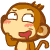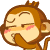Gray-Wheelwright Test | INFJ Forum

# Gray-Wheelwright Test

#### VH

##### Variable Hybrid
•Raccoon Love and NeverAmI
Your Gray-Wheelwright-Winer Type Indicator test 4 letter type is:
[SIZE=+1]
INFJ[/SIZE]

E = [SIZE=+1]4[/SIZE] S = [SIZE=+1]6[/SIZE] T = [SIZE=+1]4[/SIZE] J = [SIZE=+1]14[/SIZE] I = [SIZE=+1]6[/SIZE] N = [SIZE=+1]14[/SIZE] F = [SIZE=+1]16[/SIZE] P = [SIZE=+1]6[/SIZE]

Last edited:
Your Gray-Wheelwright-Winer Type Indicator test 4 letter type is:

INFJ

I = 10 N = 12 F = 12 J = 11
E= 0 S = 8 T = 8 P = 9

Your Gray-Wheelwright-Winer Type Indicator test 4 letter type is:
[SIZE=+1]INFJ[/SIZE]

E = [SIZE=+1]0[/SIZE] S = [SIZE=+1]7[/SIZE] T = [SIZE=+1]0[/SIZE] J = [SIZE=+1]11[/SIZE] I = [SIZE=+1]10[/SIZE] N = [SIZE=+1]13[/SIZE] F = [SIZE=+1]20[/SIZE] P = [SIZE=+1]9[/SIZE]

Nice find VH!

E = [SIZE=+1]1 I = [SIZE=+1]9[/SIZE][/SIZE]
S = [SIZE=+1]3 N = [SIZE=+1]17[/SIZE][/SIZE]
T = [SIZE=+1]16 F = [SIZE=+1]4[/SIZE][/SIZE]
J = [SIZE=+1]5 P = [SIZE=+1]15[/SIZE][/SIZE]

Nice find VH!

E = [SIZE=+1]1 I = [SIZE=+1]9[/SIZE][/SIZE]
S = [SIZE=+1]3 N = [SIZE=+1]17[/SIZE][/SIZE]
T = [SIZE=+1]16 F = [SIZE=+1]4[/SIZE][/SIZE]
J = [SIZE=+1]5 P = [SIZE=+1]15[/SIZE][/SIZE]

Thanks. And for the record, my lysdexia always reads your sig as "Peace be all up in you."

•NeverAmI
Thanks. And for the record, my lysdexia always reads your sig as "Peace be all up in you."

LOL! That is AWESOME.

INFJ

E = [SIZE=+1]0[/SIZE] S = [SIZE=+1]3[/SIZE] T = [SIZE=+1]2[/SIZE] J = [SIZE=+1]11[/SIZE] I = [SIZE=+1]10[/SIZE] N = [SIZE=+1]17[/SIZE] F = [SIZE=+1]18[/SIZE] P = [SIZE=+1]9[/SIZE]

[SIZE=+1]XNTP[/SIZE]

E = 5
I = 5

S = 3
N = 17

T = 11
F = 9

J = 6
P = 14

Your Gray-Wheelwright-Winer Type Indicator test 4 letter type is:

[SIZE=+1]INTP
[/SIZE]

E/I score E = [SIZE=+1]4[/SIZE] / I = [SIZE=+1]6[/SIZE]
S/N score S = [SIZE=+1]4 [/SIZE][SIZE=+1]/ [/SIZE]N = [SIZE=+1]16[/SIZE]
T/F score T = [SIZE=+1]12[/SIZE][SIZE=+1] / [/SIZE]F = [SIZE=+1]8[/SIZE]
J/P score J = [SIZE=+1]6 [/SIZE][SIZE=+1]/ [/SIZE]P = [SIZE=+1]14
[/SIZE]
[SIZE=+1]
-----------------------------------------------

Innnnnnnnnnnnnnnnnnnnnnnnnnteresting.[/SIZE]
[SIZE=+1][/SIZE]

[SIZE=+1]XNTP[/SIZE]

E = 5
I = 5

S = 3
N = 17

T = 11
F = 9

J = 6
P = 14

We have almost the same score!

We have almost the same score!

Looking at both of us, I think I can say we are most likely ENTP's.
Some of us are just a bit quieter than others.But we have our reasons for that.

INFP

Code:
``````E = 0   I = 10
S = 3   N = 17
T = 2   F = 18
J = 1   P = 19``````

cheers,
Ian

INFJ

Scoring higher on feeling/judging today.

E = [SIZE=+1]0[/SIZE] S = [SIZE=+1]4[/SIZE] T = [SIZE=+1]2[/SIZE] J = [SIZE=+1]15[/SIZE] I = [SIZE=+1]10[/SIZE] N = [SIZE=+1]16[/SIZE] F = [SIZE=+1]18[/SIZE] P = [SIZE=+1]5[/SIZE]

INTX

E = 1 I = 9
S = 6 N = 14
T = 15 F = 5
P = 10 J = 10

Hah, never could decide which way, always figured touch more p but that could be a lot to do with being add.

[SIZE=+1]INFJ
[/SIZE]

E / I = 0 / 10
S / N = 3 / 17
T / F = 2 / 18
J / P = 11 / 9

Many tests give me more T - otherwise no surprises.

INFJ

E = 0 S = 9 T = 5 J = 12
I = 10 N = 11 F = 15 P = 8

INTX

E = 1 I = 9
S = 6 N = 14
T = 15 F = 5
P = 10 J = 10

Hah, never could decide which way, always figured touch more p but that could be a lot to do with being add.

WEAK!

[SIZE=+1]INTP

[/SIZE]
E = [SIZE=+1]2[/SIZE]
S = [SIZE=+1]3[/SIZE]
T = [SIZE=+1]13[/SIZE]
J = [SIZE=+1]5[/SIZE]
I = [SIZE=+1]8[/SIZE]
N = [SIZE=+1]17[/SIZE]
F = [SIZE=+1]7[/SIZE]
P = [SIZE=+1]15

[/SIZE]
Cool, first test that scores my type correctly in a while.

Last edited:
E = 4
I = 6
N = 11
S = 9
F = 14
T = 6
J = 16
P = 4

INFJ

As always, J is consistently the highest score.

Last edited: